Back to Table of Contents [TOP]   Variation of H with T   From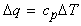one may define cp, the heat capacity at constant pressure as: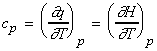..(eqn. 1) NOTE: More details on cv and cp  may be found here. The variation of H with T The variation of H with T may hence be studied by solving the differential equation above (i.e. by integration). We shall do this at two levels (1) Elementary level    Assuming cp is constant in the temperature region of interest, i.e. for T in (T1, T2),. We may re-write eqn 1. As: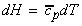i.e.:i.e.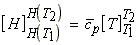i.e.: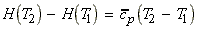(2) Higher level of complexity   If T2 is very different from T1 than the approximation that cp is constant in the temperature region of interest cannot be made. Instead we approximate cp(T) as a polynomial in T, i.e.:Note: (i) Any function can be expressed as a polynomial; (ii) For most cases, we assume thatgives a well enough description of cp. i.e.: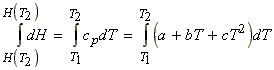i.e.: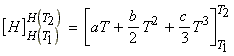i.e.:The variation of DH (or DHR) with T - applications to reactions. In analogy to eqn. 1, we may write: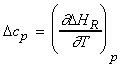(KIRCHOFF's EQN.) i.e.: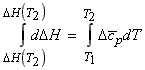which integrates to:This may be applied to compute DHR for reactions at temperature T where T 298K (i.e. not standard). In this case, cp refers to the reaction, i.e. for the reaction: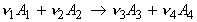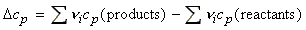Applications Example 1: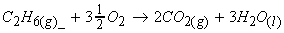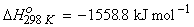(i)(ii)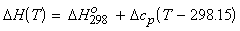Example 2: This equation could also be useful, for example, for estimating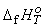for T 298K, by letting T2 = T and T1 = 298K, i.e.: